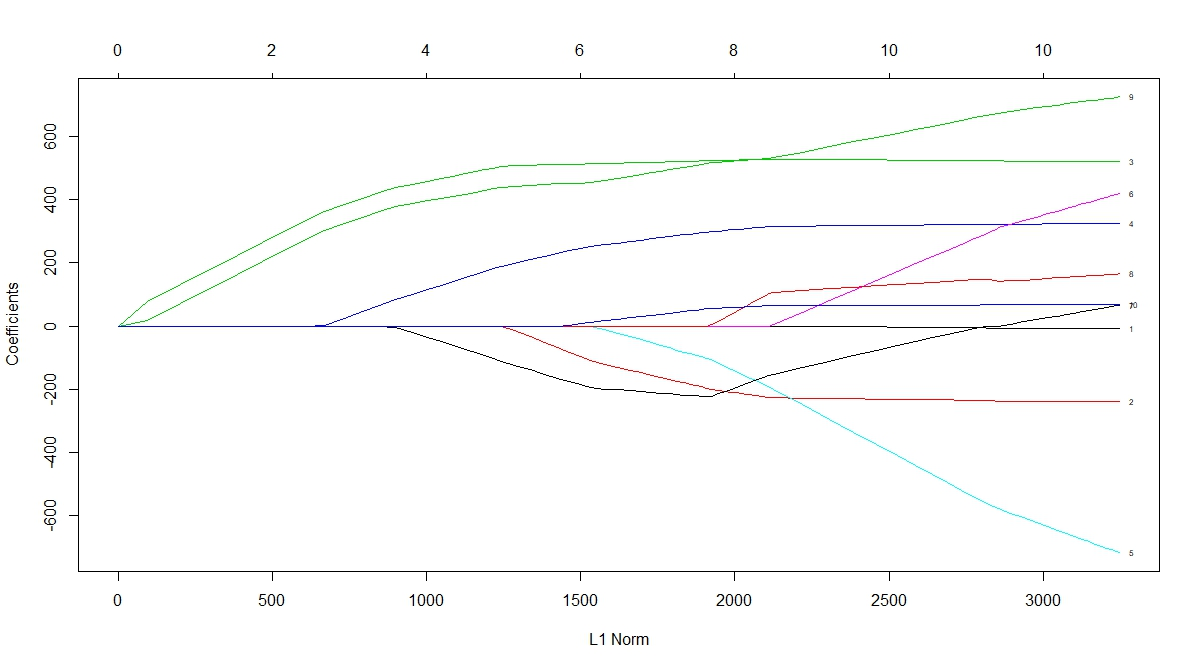Want to share your content on R-bloggers? click here if you have a blog, or here if you don't.Lease Absolute Shrinkage and Selection Operator (LASSO) performs regularization and variable selection on a given model. Depending on the size of the penalty term, LASSO shrinks less relevant predictors to (possibly) zero. Thus, it enables us to consider a more parsimonious model. In this exercise set we will use the `glmnet` package (package description: here) to implement LASSO regression in R.

Answers to the exercises are available here.

Exercise 1
Load the `lars` package and the `diabetes` dataset (Efron, Hastie, Johnstone and Tibshirani (2003) “Least Angle Regression” Annals of Statistics). This has patient level data on the progression of diabetes. Next, load the `glmnet` package that will be used to implement LASSO.

Exercise 2
The dataset has three matrices `x`, `x2` and `y`. While `x` has a smaller set of independent variables, `x2` contains the full set with quadratic and interaction terms. `y` is the dependent variable which is a quantitative measure of the progression of diabetes.
It is a good idea to visually inspect the relationship of each of the predictors with the dependent variable. Generate separate scatterplots with the line of best fit for all the predictors in `x` with `y` on the vertical axis. Use a loop to automate the process.

Exercise 3
Regress `y` on the predictors in `x` using OLS. We will use this result as benchmark for comparison.

Exercise 4
Use the `glmnet` function to plot the path of each of x’s variable coefficients against the L1 norm of the beta vector. This graph indicates at which stage each coefficient shrinks to zero.

Learn more about the glmnet package in the online course Regression Machine Learning with R. In this course you will learn how to:

• Avoid model over-fitting using cross-validation for optimal parameter selection
• Explore maximum margin methods such as best penalty of error term support vector machines with linear and non-linear kernels.
• And much more

Exercise 5
Use the `cv.glmnet` function to get the cross validation curve and the value of lambda that minimizes the mean cross validation error.

Exercise 6
Using the minimum value of lambda from the previous exercise, get the estimated beta matrix. Note that some coefficients have been shrunk to zero. This indicates which predictors are important in explaining the variation in y.

Exercise 7
To get a more parsimonious model we can use a higher value of lambda that is within one standard error of the minimum. Use this value of lambda to get the beta coefficients. Note that more coefficients are now shrunk to zero.

Exercise 8
As mentioned earlier, `x2` contains a wider variety of predictors. Using OLS, regress `y` on `x2` and evaluate results.

Exercise 9
Repeat exercise-4 for the new model.

Exercise 10
Repeat exercises 5 and 6 for the new model and see which coefficients are shrunk to zero. This is an effective way to narrow down on important predictors when there are many candidates.